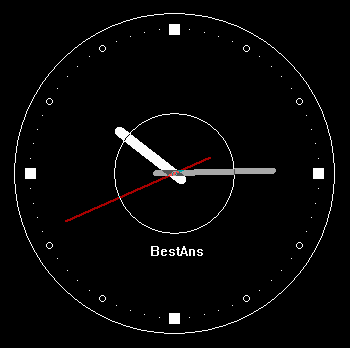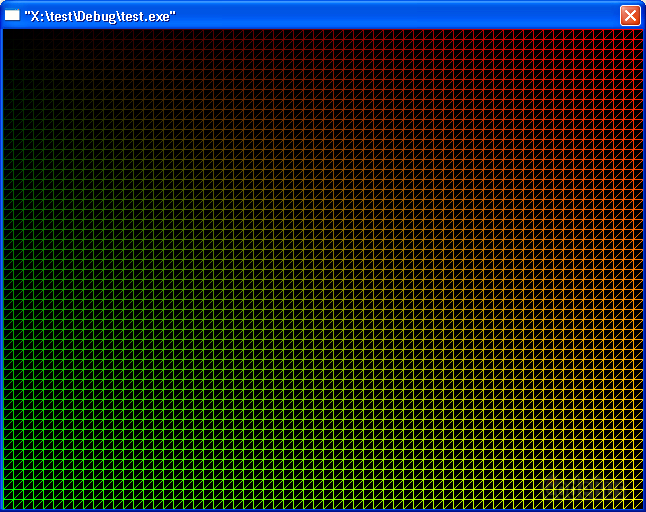# Microsoft Visual C++ 2010 学习版（推荐）

VC2010 下载地址：https://appzip

...

``````#include <graphics.h>
#include <conio.h>

int main()
{
// 初始化绘图窗口，并获取 HDC 句柄
initgraph(640, 4``````
...``````////////////////////////////////////////////
// 程序名称：钟表模拟程序(表针形式)
// 编译环境：Visual C++ 6.0，EasyX 2013白露版
// 程序编写：BestAns <BestAns@qq.com>
// 最后更新：2010-10-30
//
#include <graphics.h>
#include <conio.h>
#include <math.h>

#define	PI	3.1415926536

void DrawHand(int hour, int minute, int second)
{
double a_h``````
...

# 简单讲解

...

EasyX 库有一个获取窗口句柄的功能，很是强大，这里介绍一下。

【窗体句柄】

【函数原型】

HWND GetHWnd();

【使用句柄】

BOOL SetWindowText(HWND hWnd, LPCTSTR lpString);

hWnd: 要设置标题文

...

http://hi.baidu.com/bestans/blog/item/fb75b439404876e614cecb9f.html （已失效）

[颜色基础]

• 颜色　　直接表示　　RGB 宏标示
• 纯绿色　0x00ff00　　RGB(0, 255, 0)
• 青色　　0xffff00　　RGB(0, 255, 255)　　注：青=蓝+绿
...

getimage / putimage / loadimage / saveimage 这一组命令和 IMAGE 对象可以实现图像处理的相关功能，下面逐个介绍。
（有点类似 tc 中的 imagesize）

[加载图片]

1. 定义 IMAGE 对象
2. 读取图片至 IMAGE 对象
3. 显示 IMAGE 对象到需要的位置

``````#include <graphics.h>
#include <conio.h>

int main()
{
initgraph(640, 480);

IMAGE img;	// 定义 IMAGE 对象
...

# 一维数组

``````	int n;
int i;
for (i = 0; i < 10; i++)
n[i] = rand() % 1000;

// 按生成的顺序，逆序输出
for (i = 9; i >= 0; i--)
printf("%d\n", n[i]);

// 找出最大的
int max = -1;
for (i = 0; i < 10; i++)
{
if (n[i] > max)
max = n[i];
}

printf("最大的数字是：%d\n", ma``````
...

==本节课开始==

char c;

...

＝＝＝＝＝＝＝＝＝＝＝＝＝＝＝＝＝＝＝＝＝＝＝＝

# 一、位运算的运算法则

1. NOT

C 语言用符号 ~ 表示。

2. AND

...``````#include <graphics.h>
#include <conio.h>

// 在坐标 (x,y) 处，用颜色 c 绘制三角形
void sanjiaoxing(int x, int y, int c)
{
// 设置画线颜色
setlinecolor(c);

// 画三角形的三条边
line(x, y, x+50, y);
line(x, y, x, y+50);
line(x+``````
...

[随机函数简介]

rand()

[简单测试]

``````#include <stdio.h>
#include <stdlib.h>

int main()
{
int r;
for(int i=0; i<10; i++)
{
r = rand();
printf("%d\n", r);
}

return 0;
}``````
...

char c = _getch();

``````char c;
if (_kbhit())
c = _getch();``````

...

1. 绘制图像
2. 延时
3. 擦掉图像

``````#include <graphics.h>
#include <conio.h>

int main()
{
initgraph(640, 480);

for(int y = 0; y < 480; y++)
{
// 绘制绿色直线
setlinecolor(GREEN);
line(0, y, 639, y);

// 延时
Sleep(10);

// 绘制黑色直线（即擦掉之前``````
...

[学习目标]

1. 最简单的，来个全屏的渐变色吧，是上一课的扩展。就是需要将 0~255 的颜色和 0~479 的 y 轴对应起来
c 表示颜色，范围 0~255
y 表示y轴，范围 0~479

c / 255 = y / 479
c = y / 479 * 255 = y * 255 / 479 （先算乘法再算除法可以提高精度）

``````#include <graphics.h>
#include <conio.h>

int main()
{
initgraph(640, 480);

int c;
for(int y=0; y<48``````
...